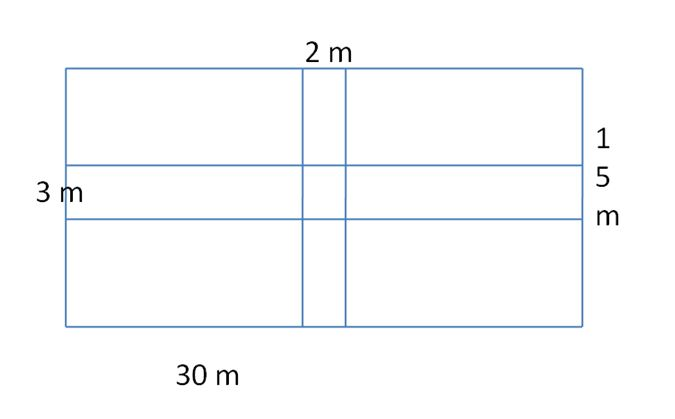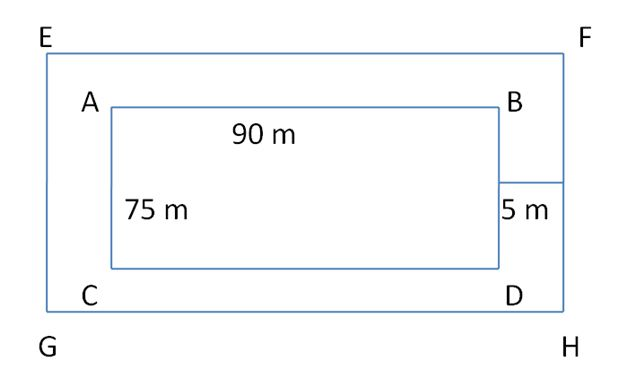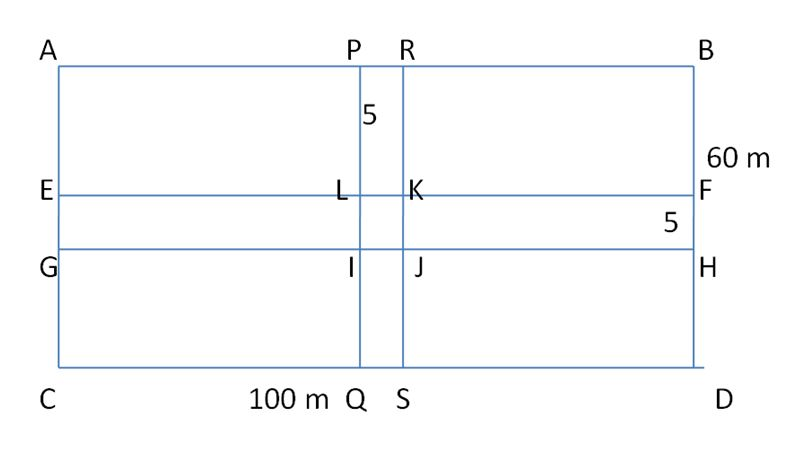# Worksheet on Area of the Path | Area of the Path Worksheet with Solutions

Are you looking for guidance on the concept of the Area of the path? Then, get the Worksheet on Area of the Path now. Here, you can get the detailed step by step explanation to solve each and every question. Solve all the questions from the Area of the Path Worksheet and re-check your answers here. By solving these problems, you will learn the concept easily and cover various models.

You can improve problem-solving skills and knowledge by solving the Area of the Path Worksheets frequently and assess your preparation standards. All questions provided here are framed by the subject experts to help the students to have a perfect knowledge of the concept.

1. A rectangular field is of dimensions 30 m × 15 m. Two paths run parallel to the sides of the rectangle through the centre of the field. The width of the longer path is 3 m and that of the shorter path is 2 m.

Find that:

(a) area of the paths

(b) area of the remaining portion of the field.

(c) cost of constructing the roads at the rate of \$10 per m².

Solution:

Given that,The dimensions of the rectangular field is 30 x 15

The width of the longer path is 3 m, the shorter path is 2 m.

The area of the rectangle = length x breadth

= 30 x 15 = 450

Area of the shorter path = 15 x 2 = 30 m

Area of the longer path = 30 x 3 = 90 m

Area of the middle path = 2 x 3 = 6

Area of paths = [Area of the shorter path] + [Area of the londer path] – [Area of the middle common path]

= 30 + 90 – 6

= 120 – 6 = 114

Area of the remaining portion of the field = The area of the rectangle – Area of path

= 450 – 114 = 336

Cost of constructing the roads at the rate of \$10 per m²

The total cost of path = 114 x 10 = \$1140

∴ The area of path is 114 m², The area of the remaining portion of the field is 336 m², Cost of constructing the roads at the rate of \$10 per m² is \$1140.

2. Find the area and perimeter of the following path.Solution:

The perimeter of the path is the sum of all faces.

So, path perimeter = 4(20) + 2(4) + 2(8)

= 80 + 8 + 16

= 104

Area of ABLK = 20 x 4 = 80

Area of EFHG = 20 x 4 = 80

Area of CDIJ = 4 x 8 = 32

Area of the path = Area of ABLK + Area of EFGH + Area of CDIJ

= 80 + 80 + 32

= 192

∴ The area of path is 192 cm², the perimeter of the path is 104 cm.

3. A garden is 90 m long and 75 m board. A path 5 m wide is to be built outside all around it along its border. Find the area of the path?

Solution:

Given that,

The garden length is 90 m, width is 75 m.Let us take ABCD as the garden.

Then, the area of path = Area of EFGH – Area of ABCD

Area of EFCGH = (90 + 5 + 5) x (75 + 5 + 5)

= 100 x 85 = 8500

Area of ABCD = 90 x 75

= 6750

Area of path = 8500 – 6750 = 1750

∴ The area of the path is 1750 m².

4. A grassy plot is 100 m x 60 m. Two cross paths each 5 m wide are constructed at right angles through the center of the field, such that each path is parallel to one of the sides of the rectangle. Find the total area used as a path.

Solution:Area of the longer path (EFGH) = 100 x 5 = 500

Area of the shorter path (PQRS) = 5 x 60 = 300

Area of the centre field (IJKL) = 5 x 5 = 25

Area of the path = (Area of the longer path + Area of the shorter path – Area of the center field)

= 500 + 300 – 25

= 800 – 25 = 775 m².

∴ The total area used as path is 775 m².

5. A floor is 15 m long and 9 m wide. A square carpet of side 5 m is laid on the floor. Find the area of the floor not carpeted?

Solution:

Given that,

The length of the floor = 15 m

The breadth of the floor = 9 m

Side of the square = 5 m

Area of the square = side²

= 5² = 5 x 5

= 25 m²

Area of the floor = length x breadth

= 15 x 9 = 135

The area of floor not carpeted = Area of the floor – Area of carpet

= 135 – 25 = 110 m²

∴ The area of the floor not carpeted is 110 m².

6. Two crossroads, each of width 10 cm, run at right angles through the center of a rectangular garden of length 600 m and breadth 300 m and parallel to its sides.

Find:

(i) The area of the garden excluding crossroads.

(ii) The area of the crossroads

(iii) Convert these areas into hectares and ares.

Solution:

Given that,

(i) Length of the garden excluding crossroads = 600 – 10 = 590 m

Breadth of the garden excluding crossroads = 300 – 10 = 290

= 590 x 290 = 171100 m²

(ii) Length of the rectangular garden = 600 m

Breadth of the rectangular garden = 300 m

The total area of the rectangular garden = (length x breadth)

= (600 x 300) = 180000 m²

Area of the crossroads = The total area of the rectangular garden – The area of the garden excluding crossroads

= 180000 – 171100

= 8900 m²

(iii) We know that,

1 square metre = 0.0001

The area of the garden excluding crossroads = 171100 x 0.0001 = 17.11 hectares

Area of the crossroads = 8900 x 0.0001 = 0.89 hectares

1 square metre = 0.01 area

The area of the garden excluding crossroads = 171100 x 0.01 = 1711 ares

Area of the crossroads = 8900 x 0.01 = 89 ares

7. A square lawn is surrounded by a path 3 m wide. If the area of the path is 360 m². Find the area of the lawn.

Solution:

Given that,

Area of the path = 360 m²

Let the side of the lawn be x m.

Then, the area of the lawn = x²

Length of outer side = (x + 6) m

Area of outer square = (x + 6)²

= x² + 12x + 36

Area of the path = Area of the outer square – Area of the lower square

360 = x² + 12x + 36 – x²

360 = 12x + 36

12x = 360 – 36

12x = 324

x = 324/12

x = 27

Side of the lawn = 27 m

Area of the lawn = 27 x 27

= 729 m².

∴ Area of the lawn = 729 m²

8. A strip of width 3 m is cut out all round from a sheet of paper with dimensions 50 m × 30 m. Find the area of the strip cut out and the area of the remaining sheet.

Solution:Given that,

Length of the sheet = 50 m

Breadth of the sheet = 30 m

Area of the sheet = 50 x 30 = 1500 m²

A strip of width 3 cm is cut out all round from a sheet of paper

Let that form a rectangle PQRS

Length of the rectangle PQRS = 50 – 3 – 3 = 50 – 6 = 44 m

Breadth of the rectangle = 30 – 3 – 3 = 30 – 6 = 24 m

Area of the rectangle PQRS = 44 x 24 = 1056 m²

Area of the strip cut out = Area of ABCD – Area of PQRS

= 1500 – 1056

= 444 m²

∴ The area of the strip cut out and the area of the remaining sheet is 444 m².

9. A path 5 m wide runs along inside a rectangular field. The length of the rectangular field is three times the breadth of the field. If the area of the path is 500 m², then find the length and breadth of the field.

Solution:

Let l be the length of the field, breadth of the field is b.

Breadth of the path = 5 m

The area of the path is 500 m².

2 x 5(l – 5) + 2 x 5(b – 5) = 500

10(l – 5) + 10(b – 5) = 500

10(l – 5 + b – 5) = 500l + b – 10 = 50

l + b = 50 + 10

l + b = 60 —- (i)

The length of the rectangular field is three times the breadth of the field

l = 3b —- (ii)

Substitute, l = 3b in equtaion (i)

3b + b = 60

4b = 60

b = 60/4

b = 15 m

Putting b = 15 in equation (i)

l + 15 = 60

l = 60 – 15

l = 45 m

∴ length and breadth of the field is 45 m, 15 m.

10. Four square flowerbeds each of the sides 4 m are dug on a piece of land 15 m long and 8 m wide. Find the area of the remaining portion of the land. Find the cost of leveling this land at a rate of \$5 per 100 cm².

Solution:

Length of the land = 15 m

Breadth of the land = 8 m

Area of the land = 15 x 8

= 120 m²

Side of the square flowerbed = 4 m

Area of 1 square bed = side²

= 4² = 4 x 4 = 16

Area of 4 square bed = 16² = 16 x 16

= 256 m²

Area of the remaining portion = 256 – 16

= 240 m²

Cost of levelling 100 cm² = \$5

Cost of levelling 0.0001 m² = \$5

Cost of levelling of 240 m² = 240 x 50000 = 12000000

Hence, the area of the remaining portion of the land is 240 m² and the cost of leveling is \$12000000.